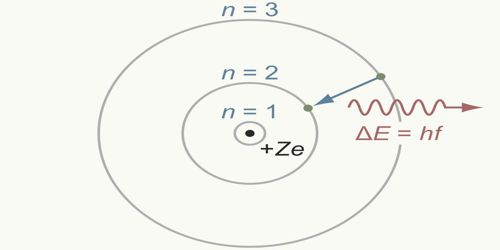Physics

# How Bohr’s Atom Model overcomes the limitations of Rutherford’s Model?In 1913, famous Dutch scientist Niels Bohr proposed this model of the atom and in 1922 he got Nobel Prize for this discovery. Bohr proposed that the laws of classical mechanics and the electromagnetism break down in case of atoms. In this model, he tried to remove the defects of Rutherford’s atom model. Rutherford proposed that electrons circled the nucleus like planets around the sun. However, his model could not explain atomic line spectra — why metals or their compounds give off characteristic colors when heated. Bohr improved Rutherford’s model by proposing that electrons traveled about the nucleus in orbits that had specific energy levels.

Bohr’s Third postulate overcomes the limitations of Rutherford’s model –

Whenever an electron jumps from a convenient orbit to another convenient orbit, then radiation or absorption of energy takes place. If the transition is from an orbit of higher energy to an orbit of lower energy then radiation of energy takes place. On the other hand, if an electron jumps from a lower orbit to a higher Electron orbit, then it absorbs energy. The amount of this radiated or absorbed energy is equal to the difference of the energies of those two orbits between which transition takes place and its value is one quantum, i.e., hv.

E = E2 ≈ E1 = hv

Here, E = radiated or absorbed energy;

E1 = energy of the lower orbit;

E2 = energy of the higher orbit;

According to the electromagnetic theory of Rutherford’s model moving charged particles always radiate energy in the form of electromagnetic radiation. Hence electrons rotating in circular orbits have centripetal acceleration and so energy should continuously be dissipated. As a resulting velocity of electron i.e., the radius of the orbit should decrease. According to Bohr’s third postulate, during the jump of an electron from one orbit to another orbit, absorption or radiation of energy is done by the electromagnetic radiation of energy. According to the wavelength that radiation can be visible light, ultraviolet rays, even X-rays. As a result, there is no effect on the circumference of the orbit. So, it can be said that the above hypotheses of Bohr’s atom model overcome the limitations of Rutherford’s model.